# Easiest colleges to get into in New York

Top 10 colleges in New York with the highest acceptance rates
What are the easiest colleges to get into in New York? We've got you covered. We've compiled a national college database and have created a list of the easiest colleges to get into in New York below. These are the easiest 4 year colleges to get into in New York that have the highest acceptance rates, and are more likely to accept low GPAs, GEDs, and everything in between. There are tons of good colleges below that accept more applicants, and we also share their average SAT and ACT scores so that you can see where you're most competitive. Read on to find out more.

## Nyack College acceptance rate

Nyack College acceptance rate is 98.4%.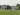The average SAT score for Nyack College is 990.

• SAT composite: 990
• SAT math: 490

The average ACT score for Nyack College is 20.

## Culinary Institute of America acceptance rate

Culinary Institute of America acceptance rate is 97.2%.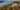The average SAT score for Culinary Institute of America is 1077.

• SAT composite: 1077
• SAT math: 530

The average ACT score for Culinary Institute of America is 22.

## D'Youville College acceptance rate

D'Youville College acceptance rate is 96.8%.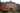The average SAT score for D'Youville College is 1113.

• SAT composite: 1113
• SAT math: 560

The average ACT score for D'Youville College is 23.

## SUNY Cobleskill acceptance rate

SUNY Cobleskill acceptance rate is 94%.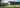The average SAT score for SUNY Cobleskill is 970.

• SAT composite: 970
• SAT math: 480

The average ACT score for SUNY Cobleskill is 20.

## Mount Saint Mary College acceptance rate

Mount Saint Mary College acceptance rate is 93.8%.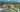The average SAT score for Mount Saint Mary College is 1091.

• SAT composite: 1091
• SAT math: 540

The average ACT score for Mount Saint Mary College is 21.

## Keuka College acceptance rate

Keuka College acceptance rate is 93.7%.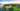The average SAT score for Keuka College is 1040.

• SAT composite: 1040
• SAT math: 520

The average ACT score for Keuka College is 20.

## Cazenovia College acceptance rate

Cazenovia College acceptance rate is 92.4%.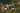The average SAT score for Cazenovia College is 1010.

• SAT composite: 1010
• SAT math: 500

The average ACT score for Cazenovia College is 22.

## Manhattanville College acceptance rate

Manhattanville College acceptance rate is 89.9%.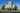The average SAT score for Manhattanville College is 1119.

• SAT composite: 1119
• SAT math: 560

The average ACT score for Manhattanville College is 22.

## Hartwick College acceptance rate

Hartwick College acceptance rate is 88.6%.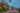The average SAT score for Hartwick College is 1010.

• SAT composite: 1010
• SAT math: 510

The average ACT score for Hartwick College is 24.

## Iona College acceptance rate

Iona College acceptance rate is 88.2%.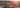The average SAT score for Iona College is 1090.

• SAT composite: 1090
• SAT math: 540